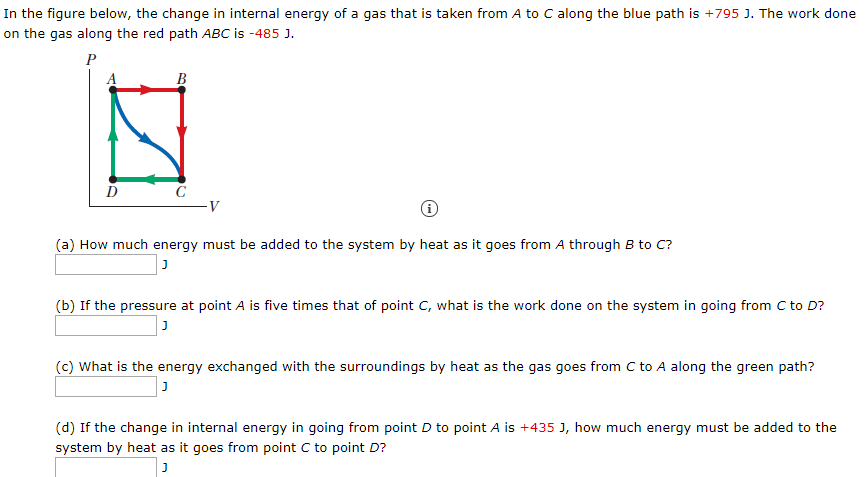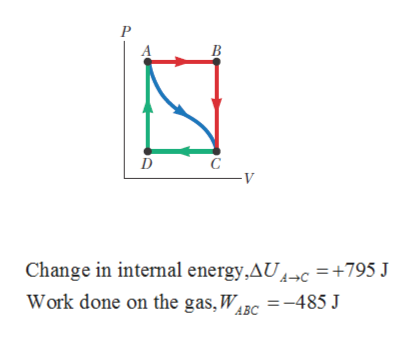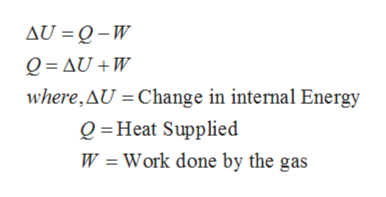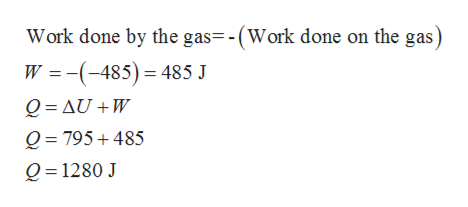# In the figure below, the change in internal energy of a gas that is taken from A to C along the blue path is +795 J. The work doneon the gas along the red path ABC is -485 J.B(a) How much energy must be added to the system by heat as it goes from A through B to C?(b) If the pressure at point A is five times that of point C, what is the work done on the system in going from C to D?(c) What is the energy exchanged with the surroundings by heat as the gas goes from C to A along the green path?(d) If the change in internal energy in going from point D to point A is +435 J, how much energy must be added to thesystem by heat as it goes from point C to point D?

Question
36 viewshelp_outlineImage TranscriptioncloseIn the figure below, the change in internal energy of a gas that is taken from A to C along the blue path is +795 J. The work done on the gas along the red path ABC is -485 J. B (a) How much energy must be added to the system by heat as it goes from A through B to C? (b) If the pressure at point A is five times that of point C, what is the work done on the system in going from C to D? (c) What is the energy exchanged with the surroundings by heat as the gas goes from C to A along the green path? (d) If the change in internal energy in going from point D to point A is +435 J, how much energy must be added to the system by heat as it goes from point C to point D? fullscreen
check_circle

Step 1

Given,help_outlineImage TranscriptioncloseChange in internal energy,AU4¬c =+795 J Work done on the gas, W =-485 J ABC fullscreen
Step 2

Part (a):

By using 1st law of thermodynamics we can get,help_outlineImage TranscriptioncloseAU = Q-W Q = AU +W where, AU = Change in internal Energy Q =Heat Supplied W = Work done by the gas fullscreen
Step 3

Since the work is done on the gas which is negative so work done by the gas will ...help_outlineImage TranscriptioncloseWork done by the gas=-(Work done on the gas) W =-(-485)= 485 J Q = AU +W Q = 795+ 485 Q= 1280 J fullscreen

### Want to see the full answer?

See Solution

#### Want to see this answer and more?

Solutions are written by subject experts who are available 24/7. Questions are typically answered within 1 hour.*

See Solution
*Response times may vary by subject and question.
Tagged in

### Thermodynamics